Class 9
Important Questions for Exam - Class 9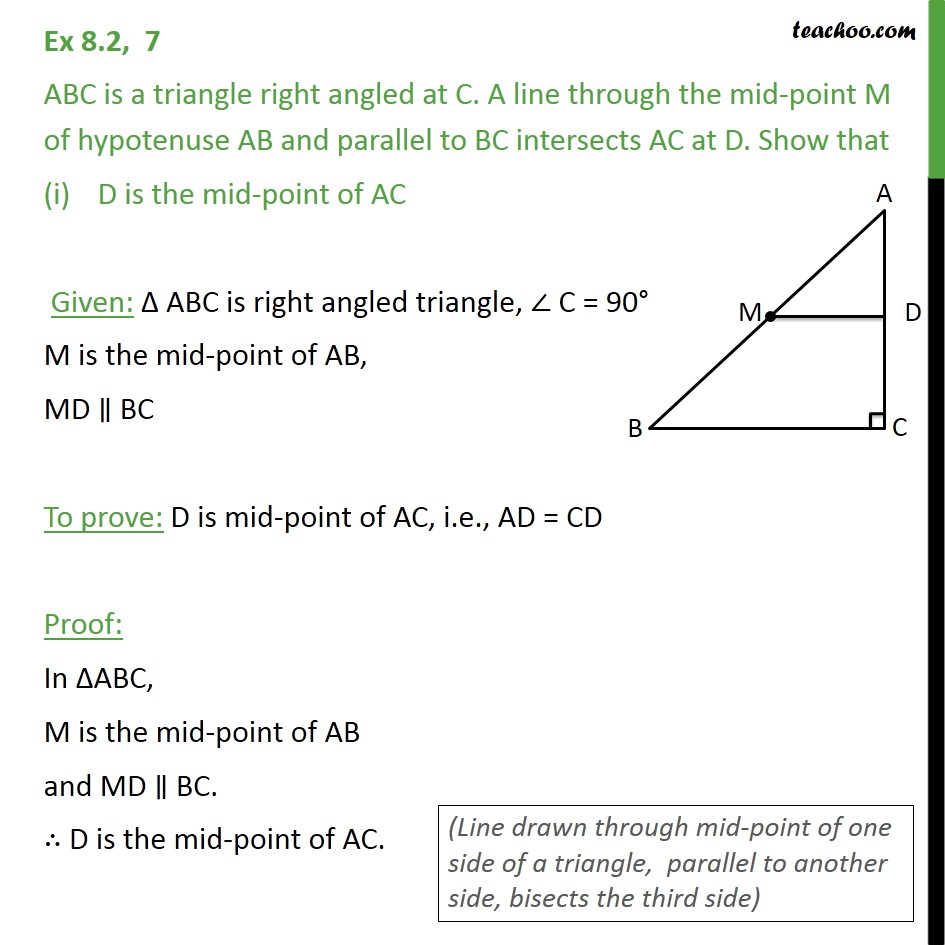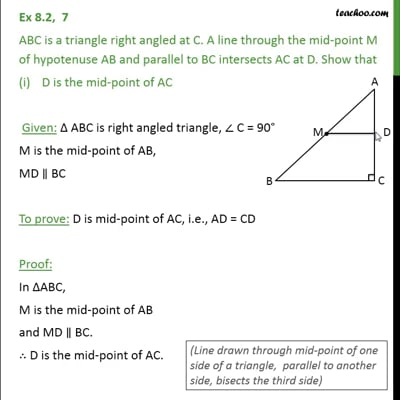This video is only available for Teachoo black users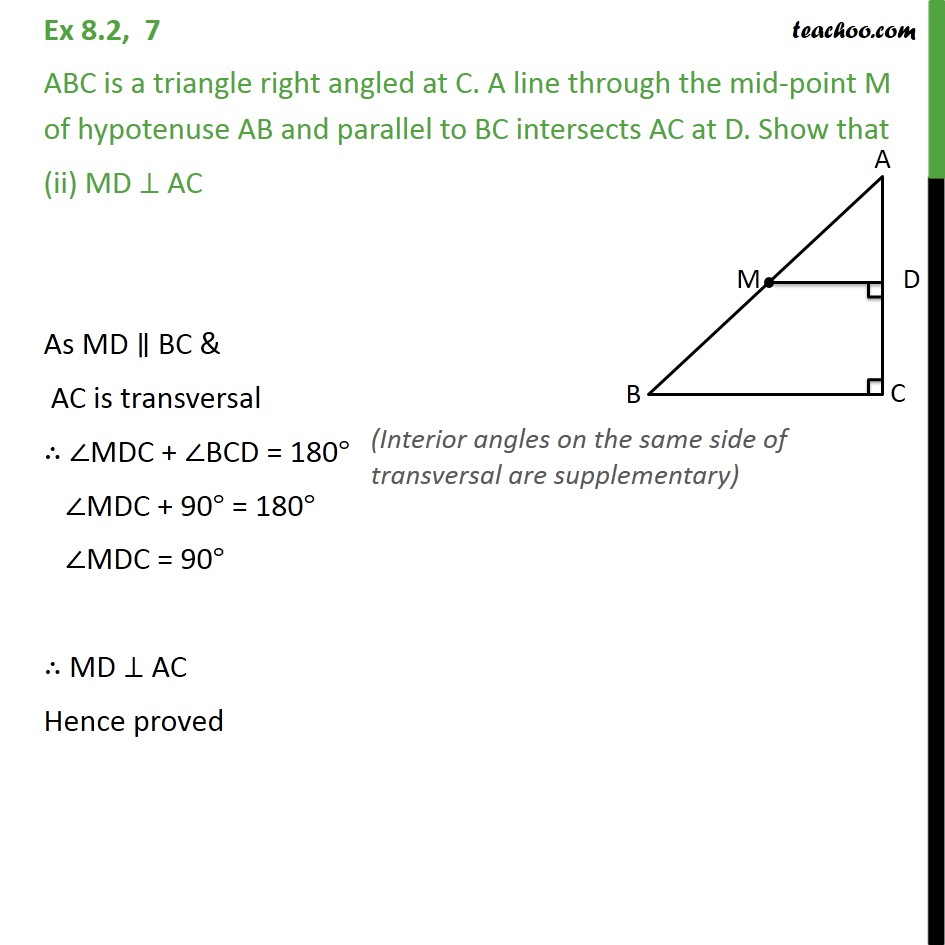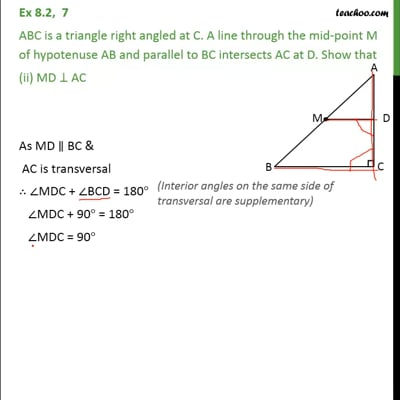This video is only available for Teachoo black users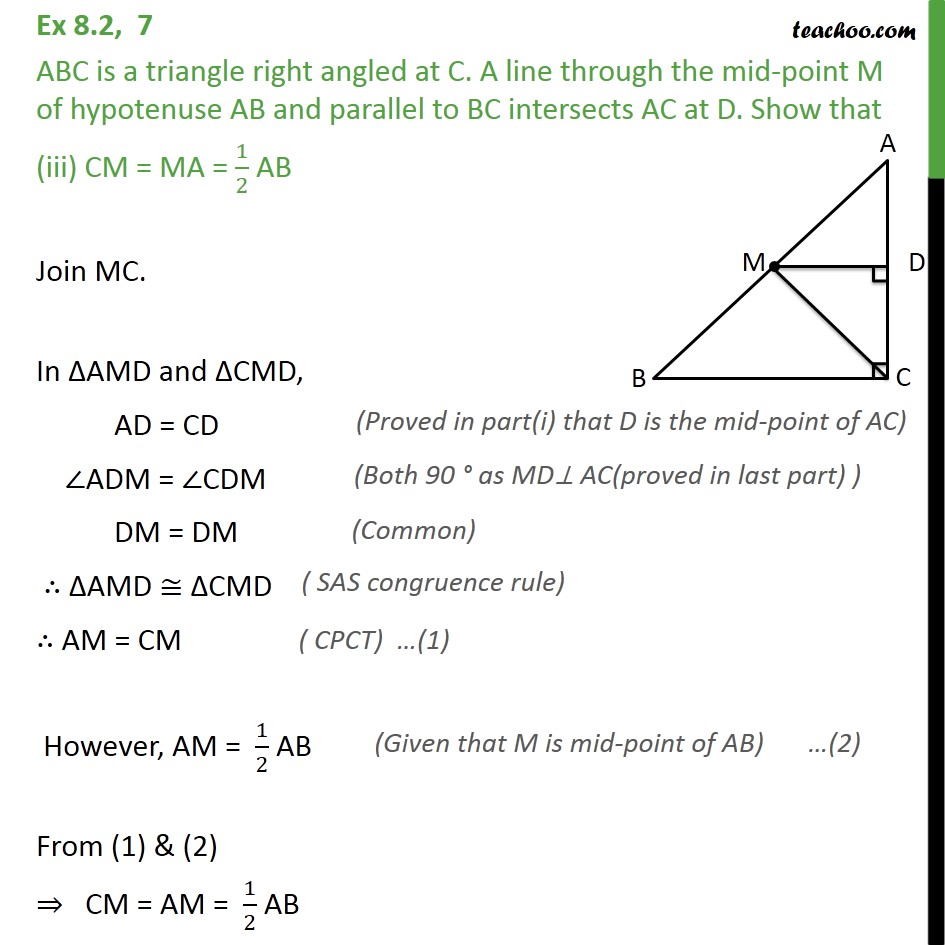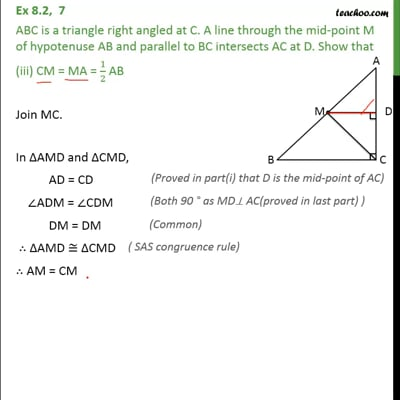This video is only available for Teachoo black users

### Transcript

Ex 8.2, 7 ABC is a triangle right angled at C. A line through the mid-point M of hypotenuse AB and parallel to BC intersects AC at D. Show that D is the mid-point of AC Given: ABC is right angled triangle, C = 90 M is the mid-point of AB, MD BC To prove: D is mid-point of AC, i.e., AD = CD Proof: In ABC, M is the mid-point of AB and MD BC. D is the mid-point of AC. Ex 8.2, 7 ABC is a triangle right angled at C. A line through the mid-point M of hypotenuse AB and parallel to BC intersects AC at D. Show that (ii) MD AC As MD BC & AC is transversal MDC + BCD = 180 MDC + 90 = 180 MDC = 90 MD AC Hence proved Ex 8.2, 7 ABC is a triangle right angled at C. A line through the mid-point M of hypotenuse AB and parallel to BC intersects AC at D. Show that (iii) CM = MA = 1/2 AB Join MC. In AMD and CMD, AD = CD ADM = CDM DM = DM AMD CMD AM = CM However, AM = 1/2 AB From (1) & (2) CM = AM = 1/2 AB